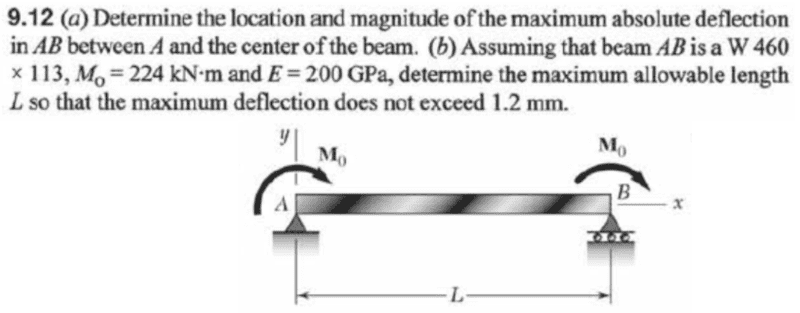# Beam deflection

## Homework Statement## Homework Equations

Singularity equations for beam deflection
$$\sum M_B=0=-M_0-R_AL-M_0$$
$$R_A=-\frac{2M_0}{L}$$

## The Attempt at a Solution

I know how to use singularity equations and all that but my problem is calculating the force especially when moment is involved. For example, from the above why is the sum of moment about B not equal to M0+RAL instead?
I guess I still don't really understand the conventions that are used in material science.

And for the moment applied on the beam as shown, is the force due to that moment acting downward or upward on that point?

In addition, how do I know that the moment(shown using the above circular arrow) is about what axix/reference? Cause the object will rotate in the opposite direction if the wrong reference is used. And to know what direction of its moment, I need to know the direction of the force at that point causing that moment, bringing me back to the question in the previous paragraph.

Thanks.

Last edited:

PhanthomJay
Homework Helper
Gold Member

## Homework Statement

View attachment 230499

## Homework Equations

Singularity equations for beam deflection
$$\sum M_B=0=-M_0-R_AL-M_0$$
$$R_A=-\frac{2M_0}{L}$$

## The Attempt at a Solution

I know how to use singularity equations and all that but my problem is calculating the force especially when moment is involved.
I think you mean calculating the reaction forces at the supports.
For example, from the above why is the sum of moment about B not equal to M0+RAL instead?
It is equal to 2 M_o + R_a(L), assuming cw moments are positive and R_a acts upward. In your first attempt, you assumed that clockwise moments are negative, and you assumed that R_a acts upward, producing a clockwise or negative moment about B. This is Ok. But when you solved R_a and got a negative value for it, that means you assumed the wrong direction for it, so R_a acts downward on the beam. R_b must act upward, for equilibrium
The applied moments are sometimes called couples, and act about the z axis. You might think of them as a torque tightening a bolt at the face of the beam at the far ends.
I guess I still don't really understand the conventions that are used in material science.
Moments convention can be positive clockwise or negative clockwise, your choice. In engineering , cw moments are most often considered positive, by convention.
And for the moment applied on the beam as shown, is the force due to that moment acting downward or upward on that point?
the direction of the reaction forces at the supports as caused by the moments has already been addressed
In addition, how do I know that the moment(shown using the above circular arrow) is about what axix/reference? Cause the object will rotate in the opposite direction if the wrong reference is used. And to know what direction of its moment, I need to know the direction of the force at that point causing that moment, bringing me back to the question in the previous paragraph.

Thanks.
by the right hand rule, the moments shown act about the z axis, and cause bending in the xy plane. Often an isometric 3D sketch is required for moments applied about other axes. The reaction force does not cause the moment, it's just the opposite. Sometimes the applied moments are given as force couples...2 equal and opposite forces a perpendicular distance apart. Don't confuse these forces with the support reaction forces.

•TimeRip496
I think you mean calculating the reaction forces at the supports. It is equal to 2 M_o + R_a(L), assuming cw moments are positive and R_a acts upward. In your first attempt, you assumed that clockwise moments are negative, and you assumed that R_a acts upward, producing a clockwise or negative moment about B. This is Ok. But when you solved R_a and got a negative value for it, that means you assumed the wrong direction for it, so R_a acts downward on the beam. R_b must act upward, for equilibrium The applied moments are sometimes called couples, and act about the z axis. You might think of them as a torque tightening a bolt at the face of the beam at the far ends. Moments convention can be positive clockwise or negative clockwise, your choice. In engineering , cw moments are most often considered positive, by convention. the direction of the reaction forces at the supports as caused by the moments has already been addressedby the right hand rule, the moments shown act about the z axis, and cause bending in the xy plane. Often an isometric 3D sketch is required for moments applied about other axes. The reaction force does not cause the moment, it's just the opposite. Sometimes the applied moments are given as force couples...2 equal and opposite forces a perpendicular distance apart. Don't confuse these forces with the support reaction forces.
Thanks a lot!!